# RD Sharma Solutions for Class 8 Maths Chapter 2 - Powers

RD Sharma Solutions for Class 8 Maths Chapter 2- Powers are available here. Our expert faculty team has prepared solutions in order to help you with your exam preparation to acquire good marks in Maths. RD Sharma Solutions book for Class 8 Maths comes in very handy at this point. Our solution module utilizes numerous shortcut tips and practical examples to explain all the exercise questions in a simple and easily understandable language. If you wish to secure an excellent score, solving RD Sharma Class 8 Solutions is essential. These solutions will help you in obtaining knowledge and strong command over the subject.

This chapter mainly deals with laws of integral (both positive and negative) exponents of rational numbers. In order to help you understand and solve the problems, we at BYJU’S have prepared the RD Sharma Maths Solutions for Class 8 Chapter 2 where the problems are solved in detail. Download pdf of Class 8 Chapter 2 in their respective links.

## Download PDF of RD Sharma Solutions for Class 8 Maths Chapter 2 – Powers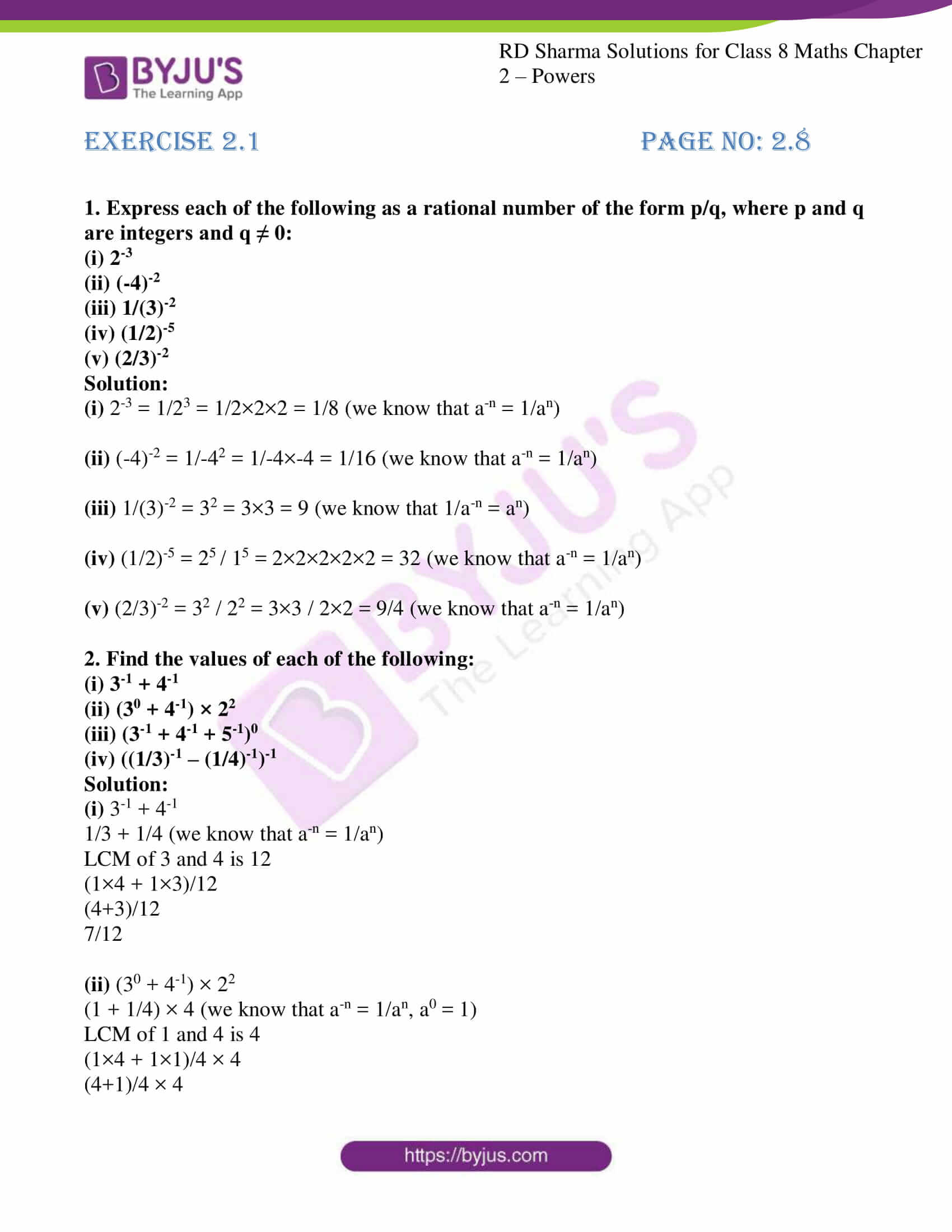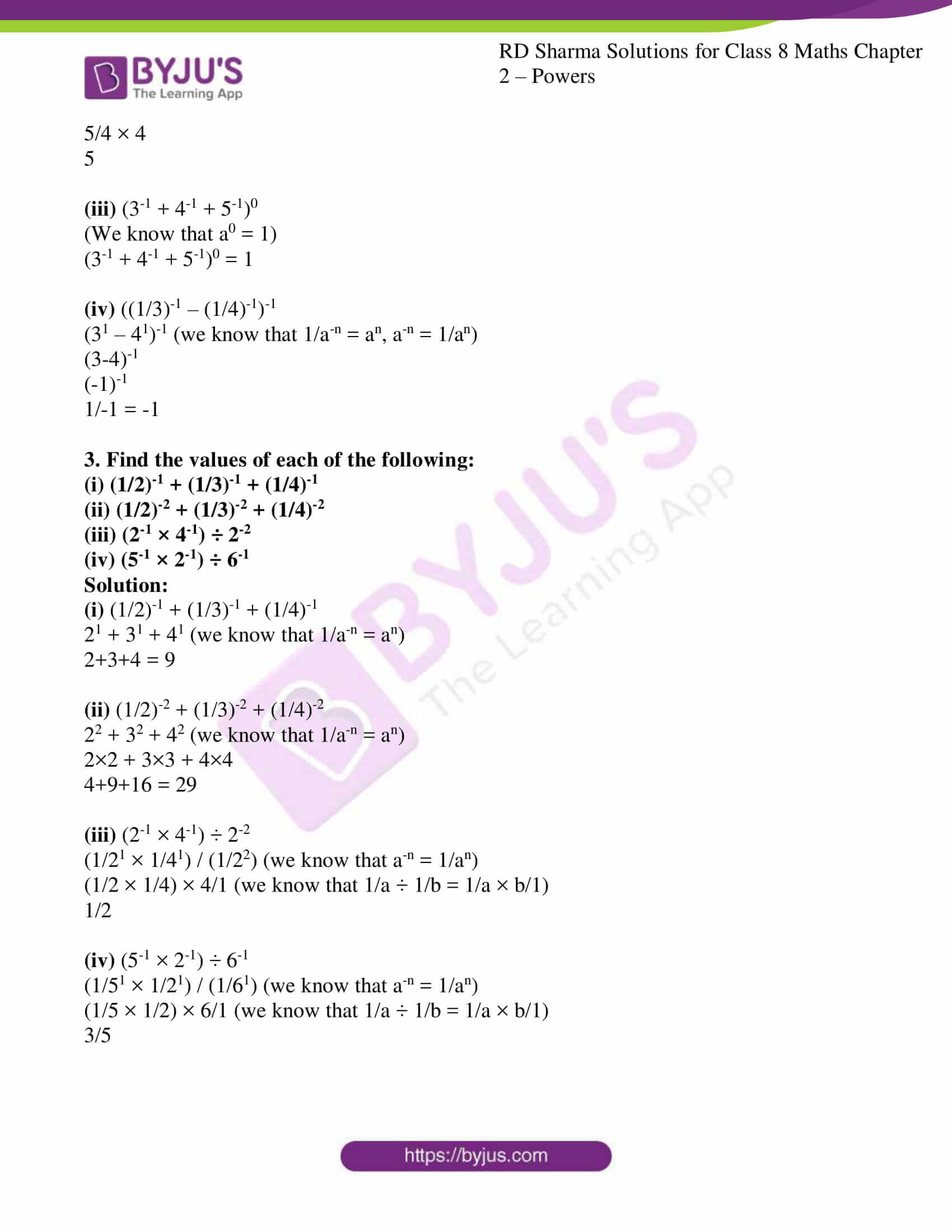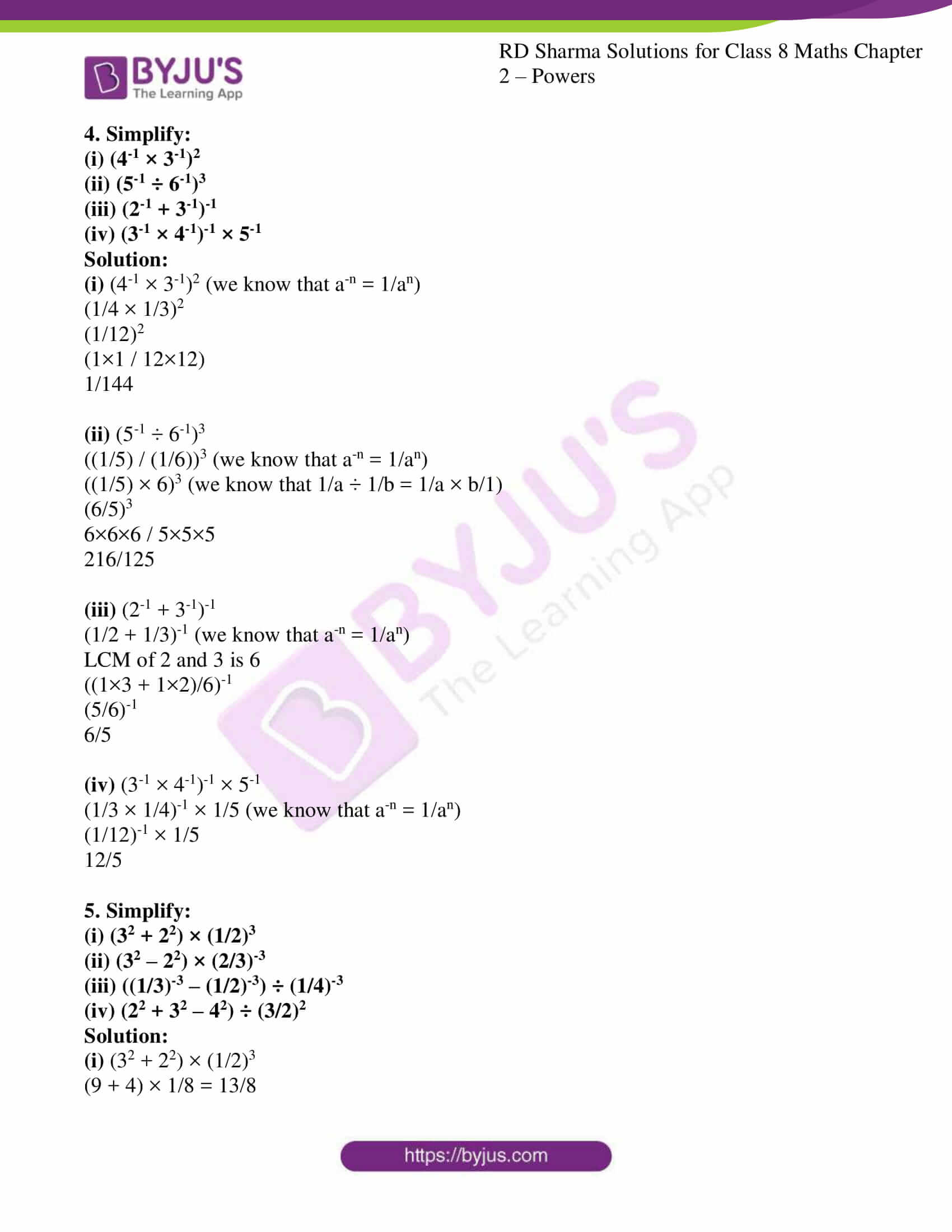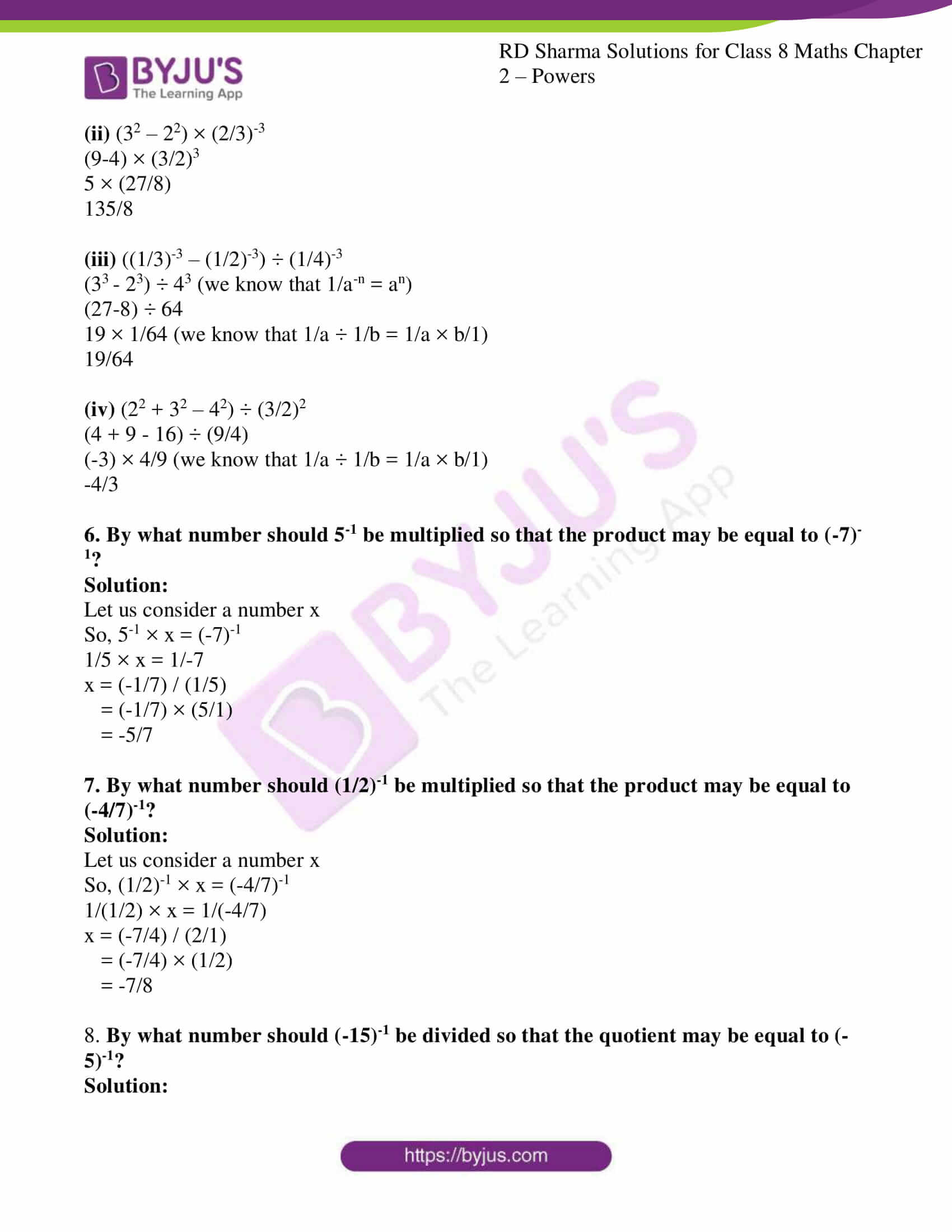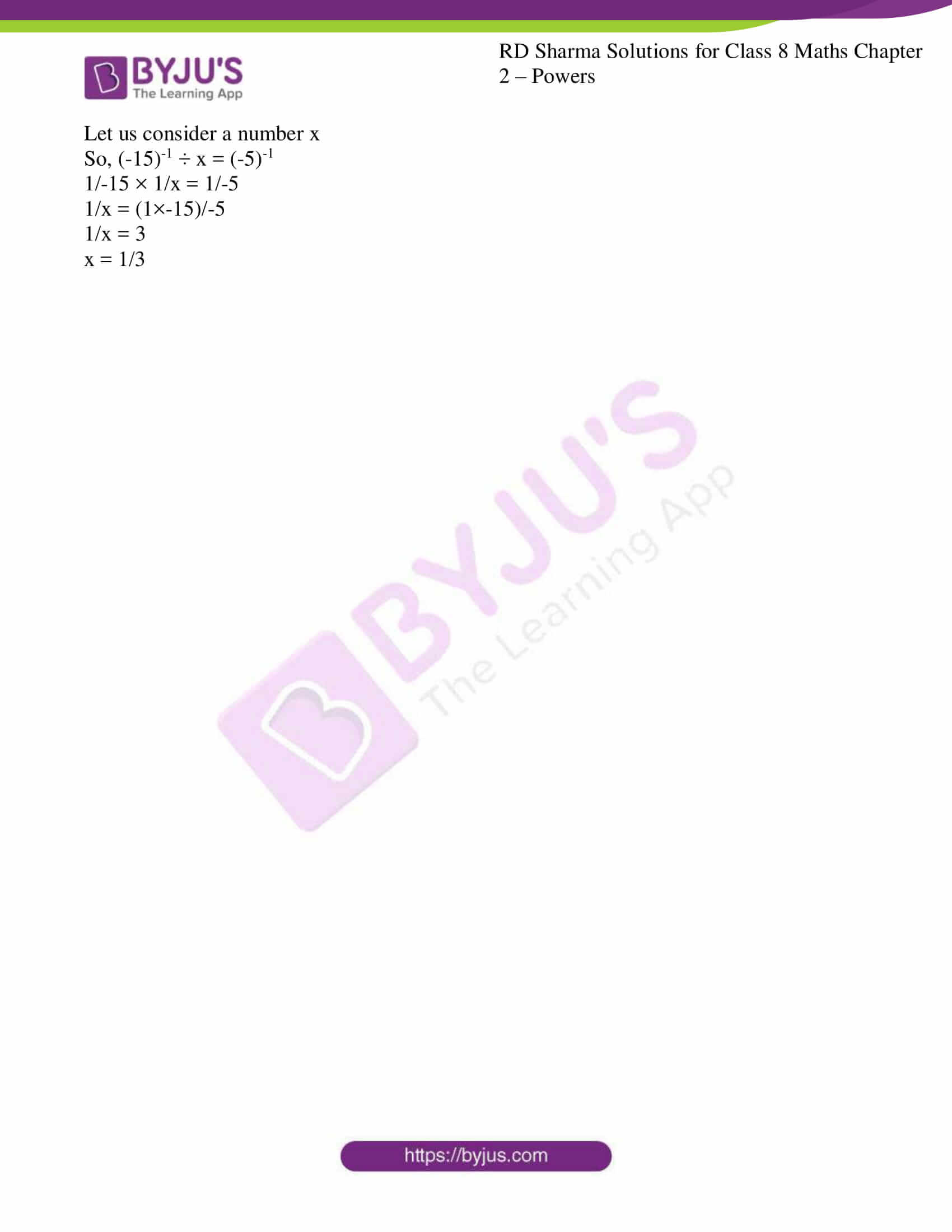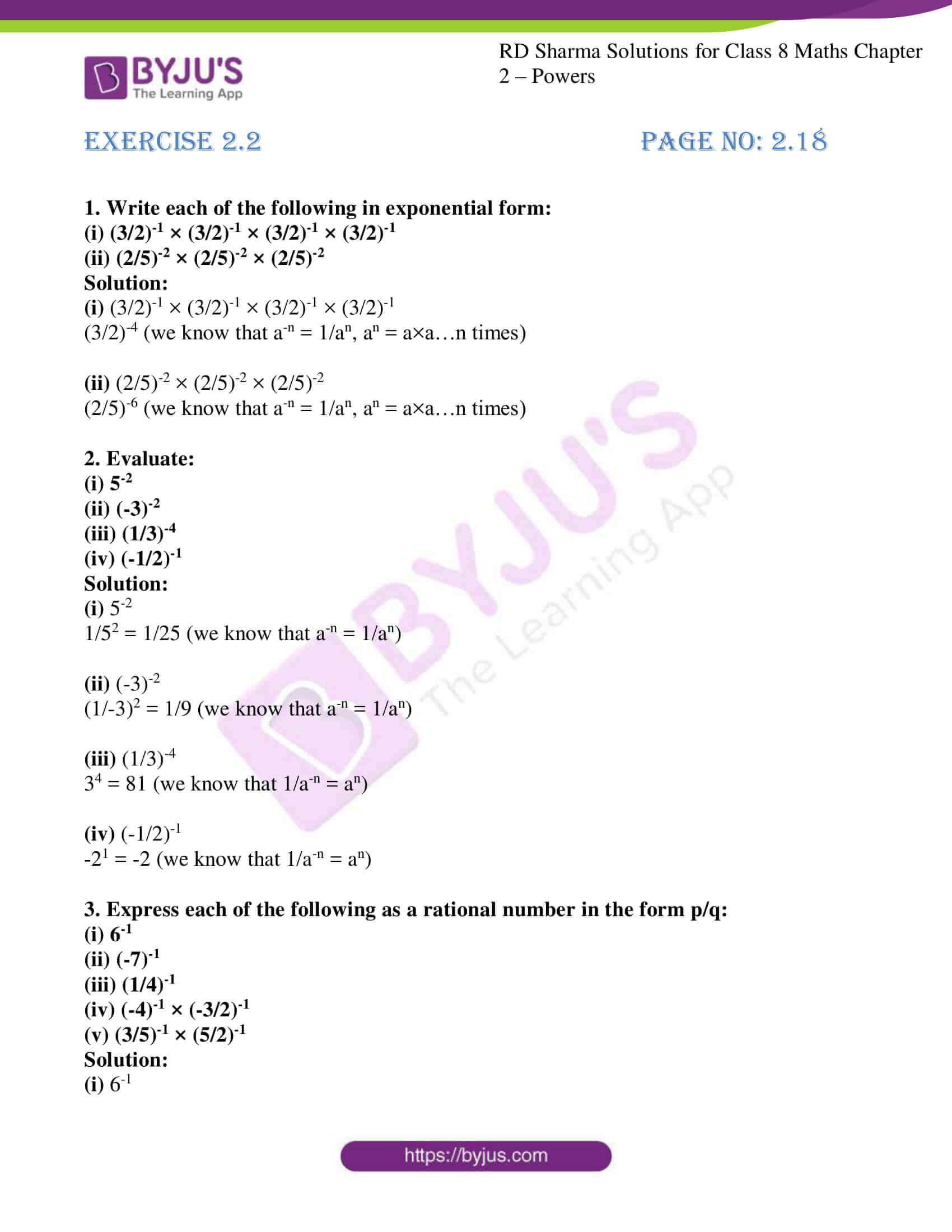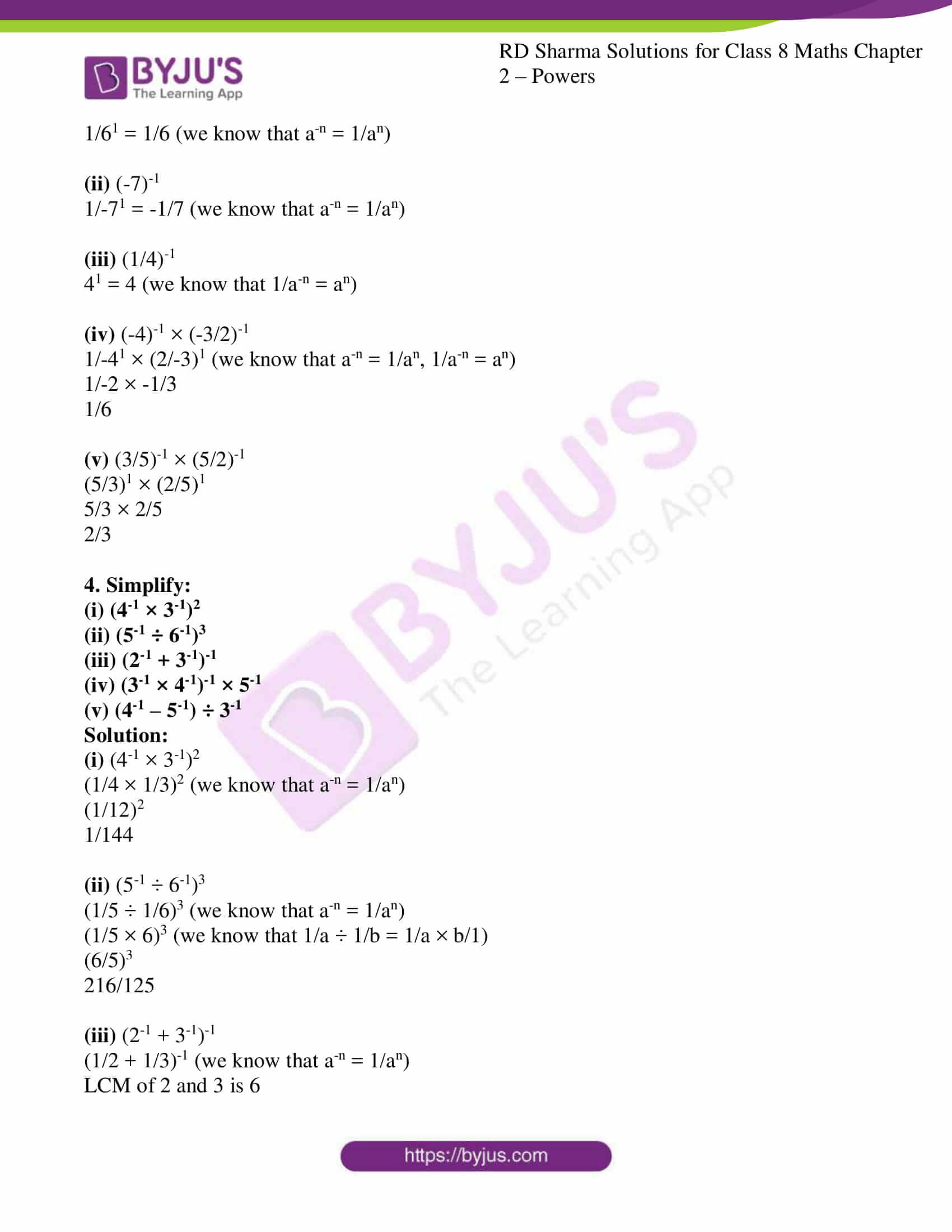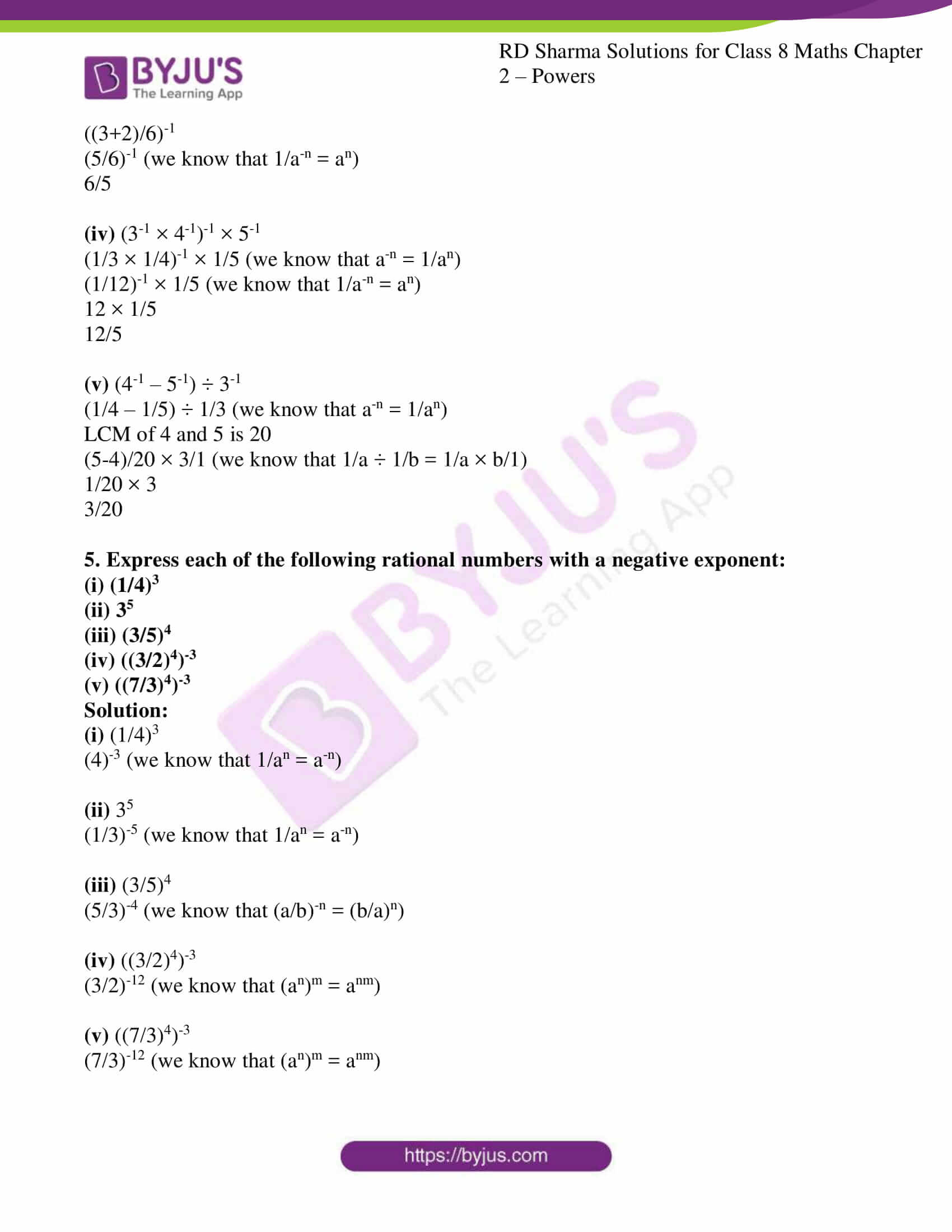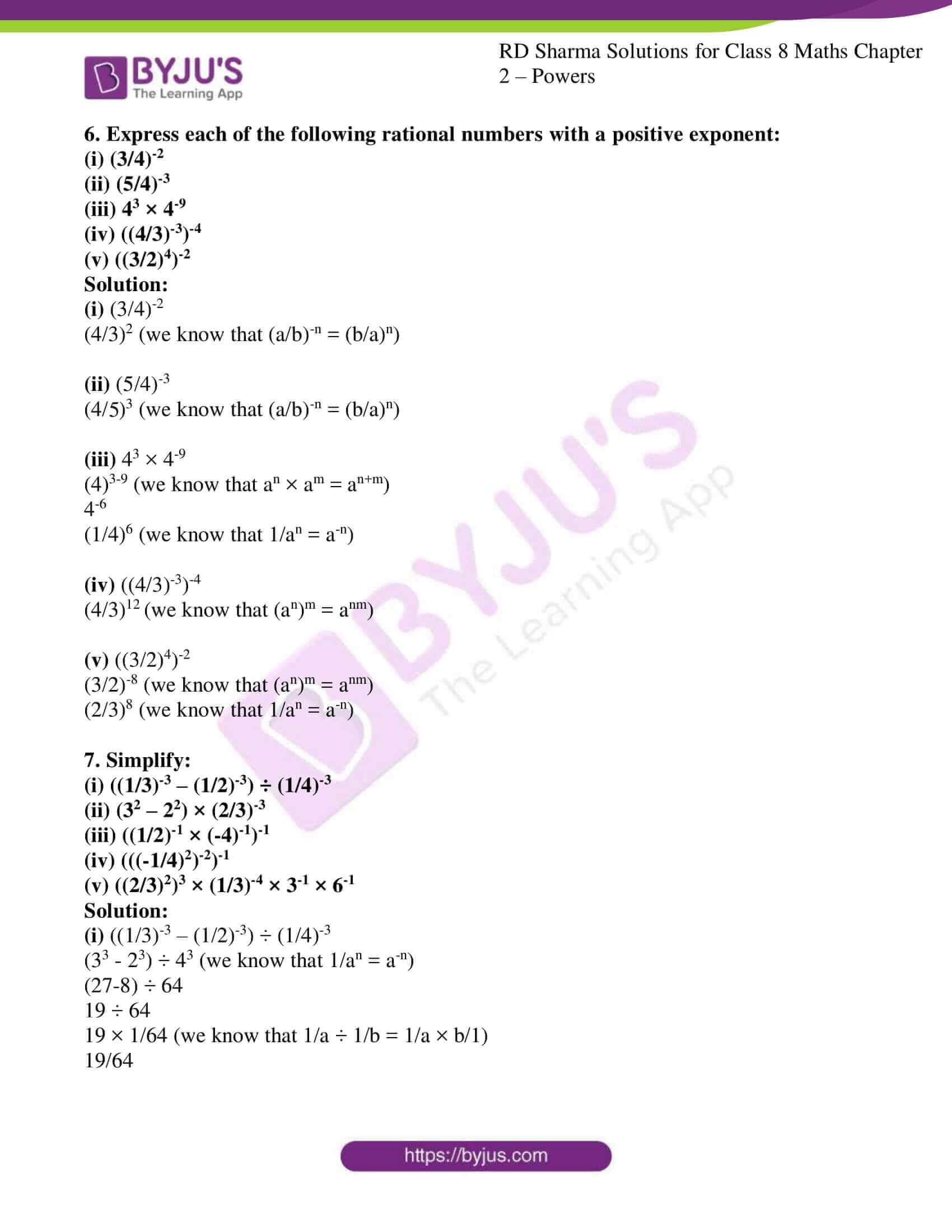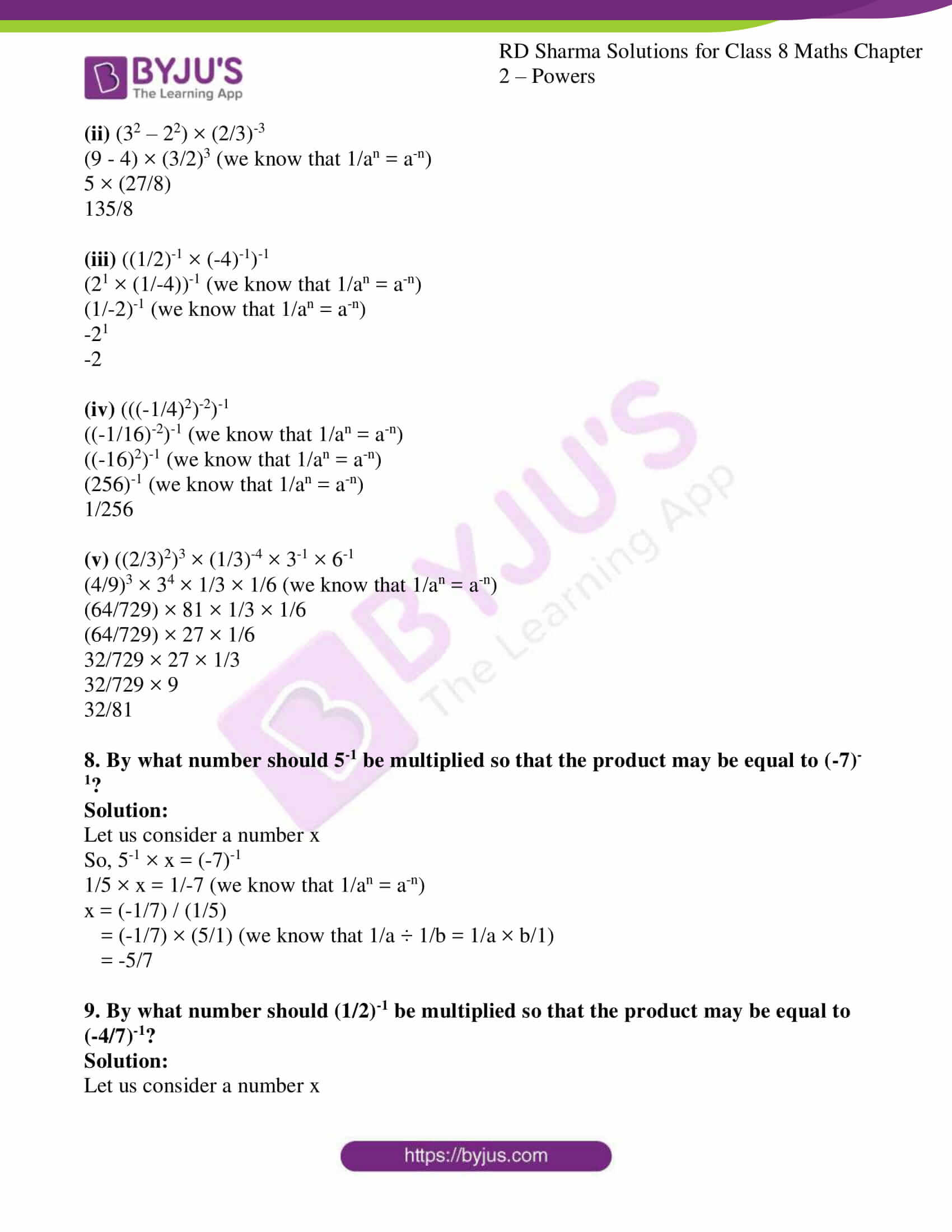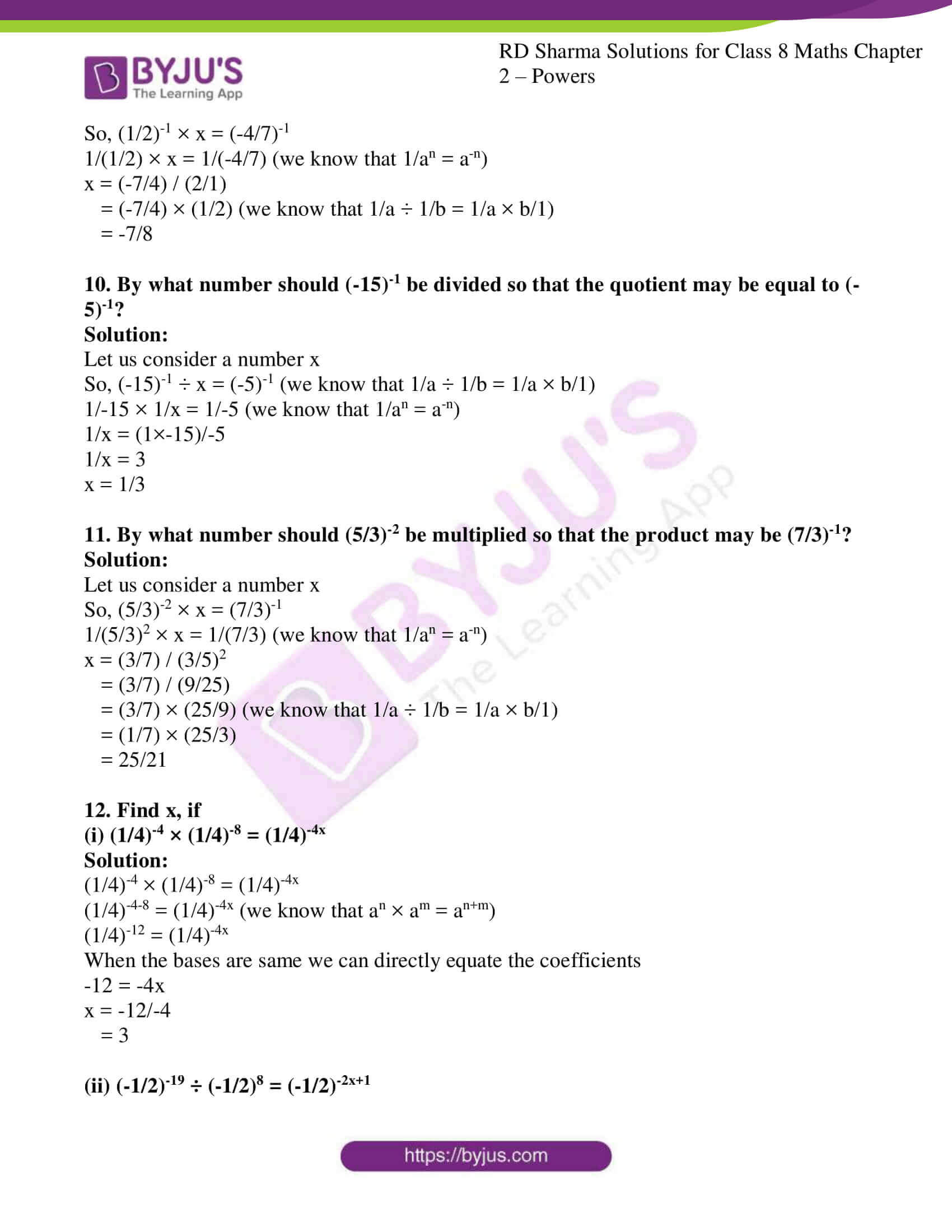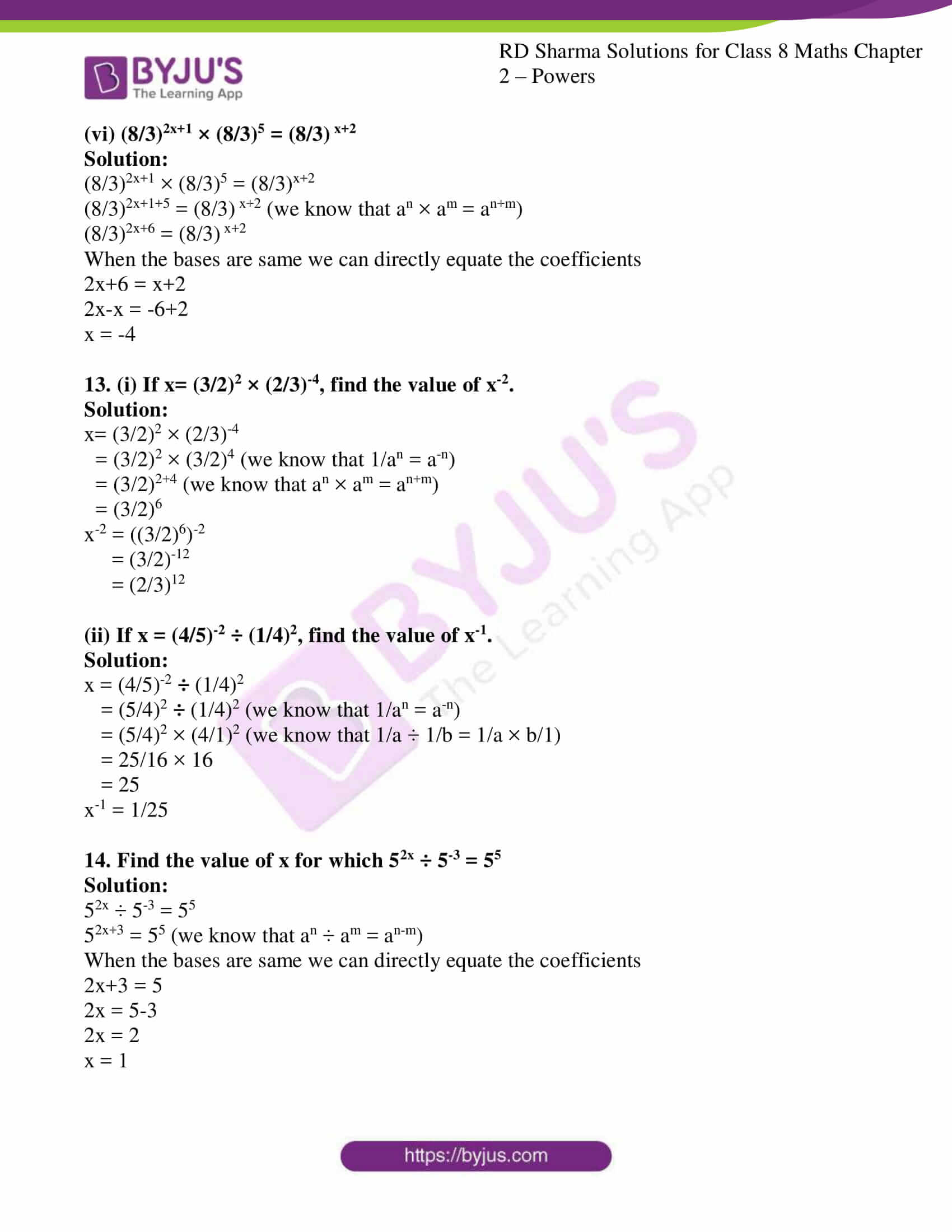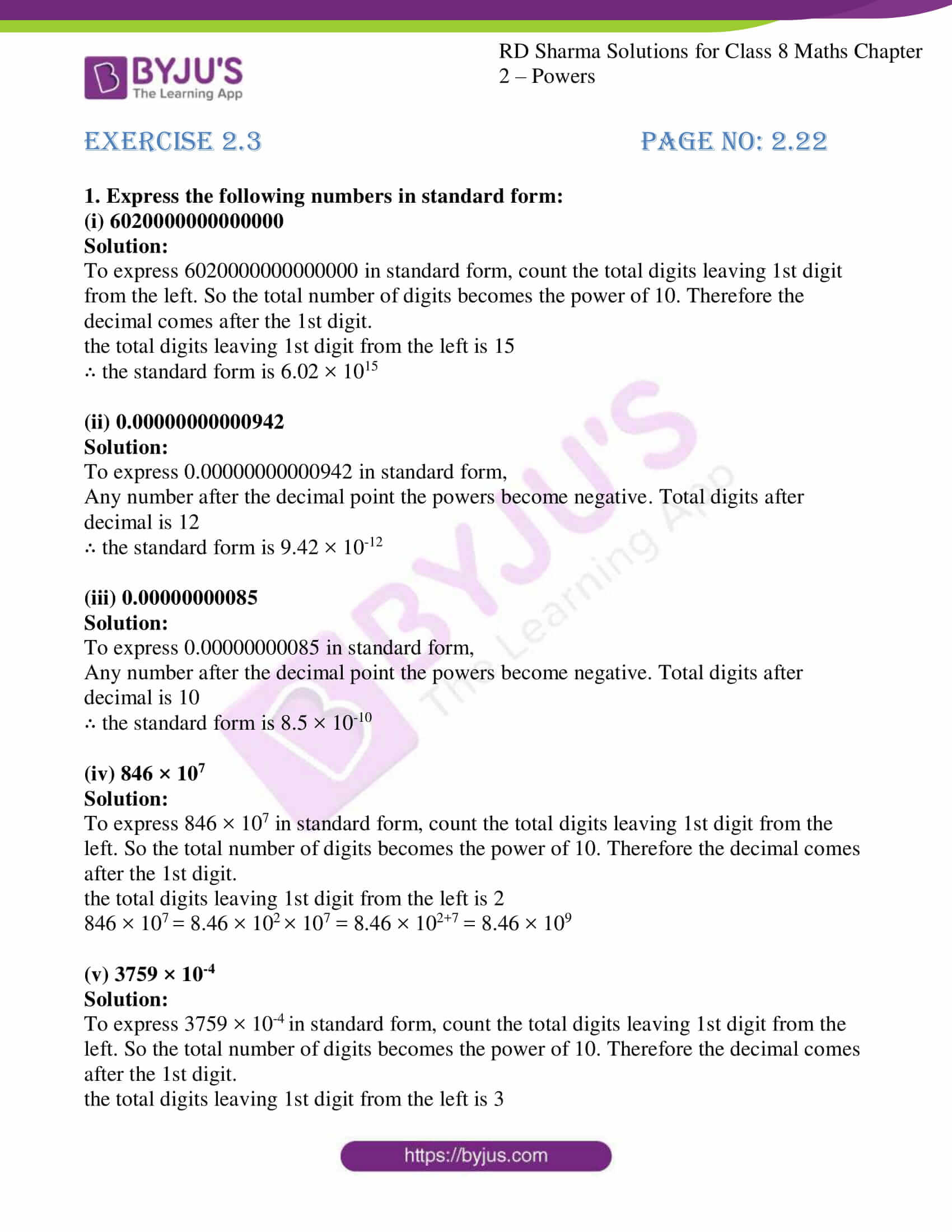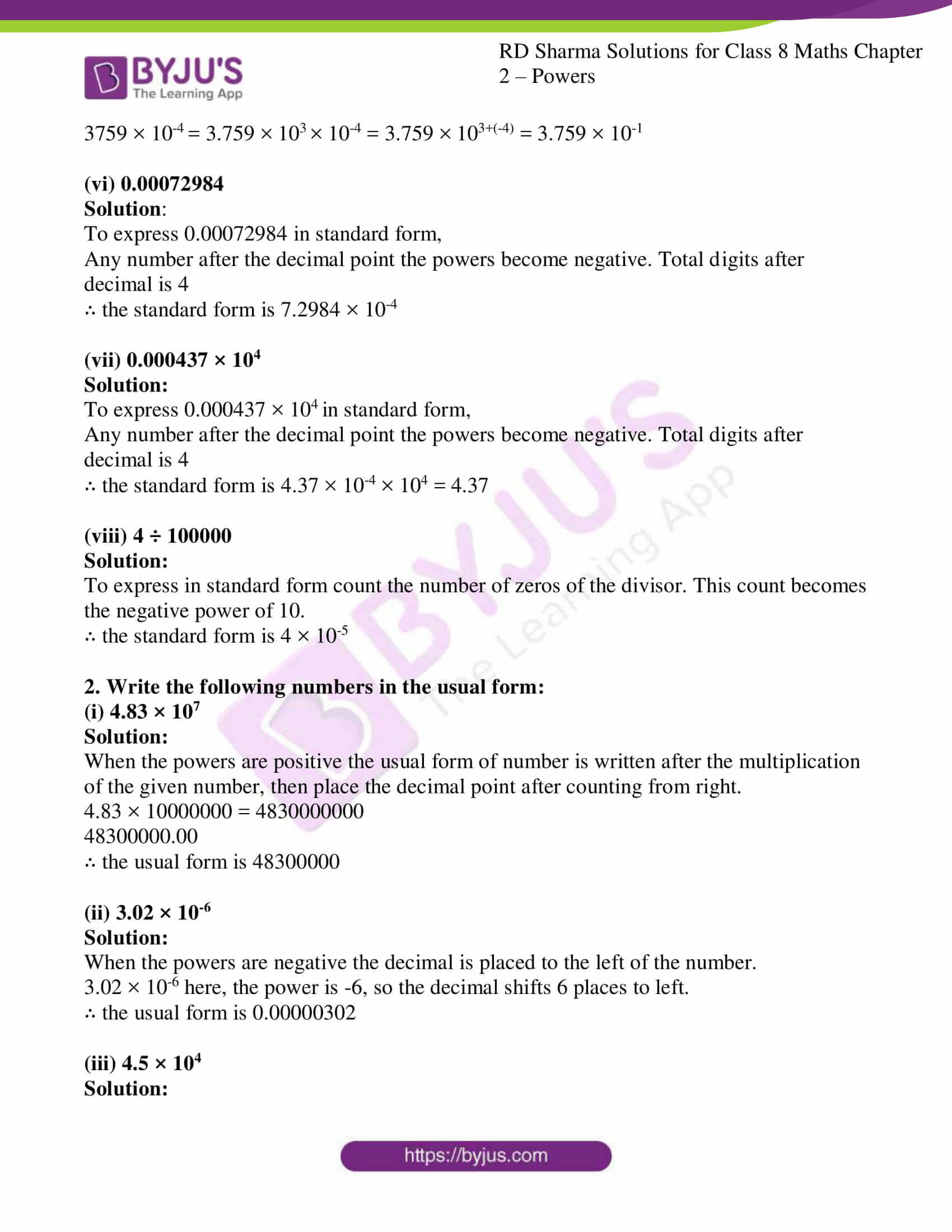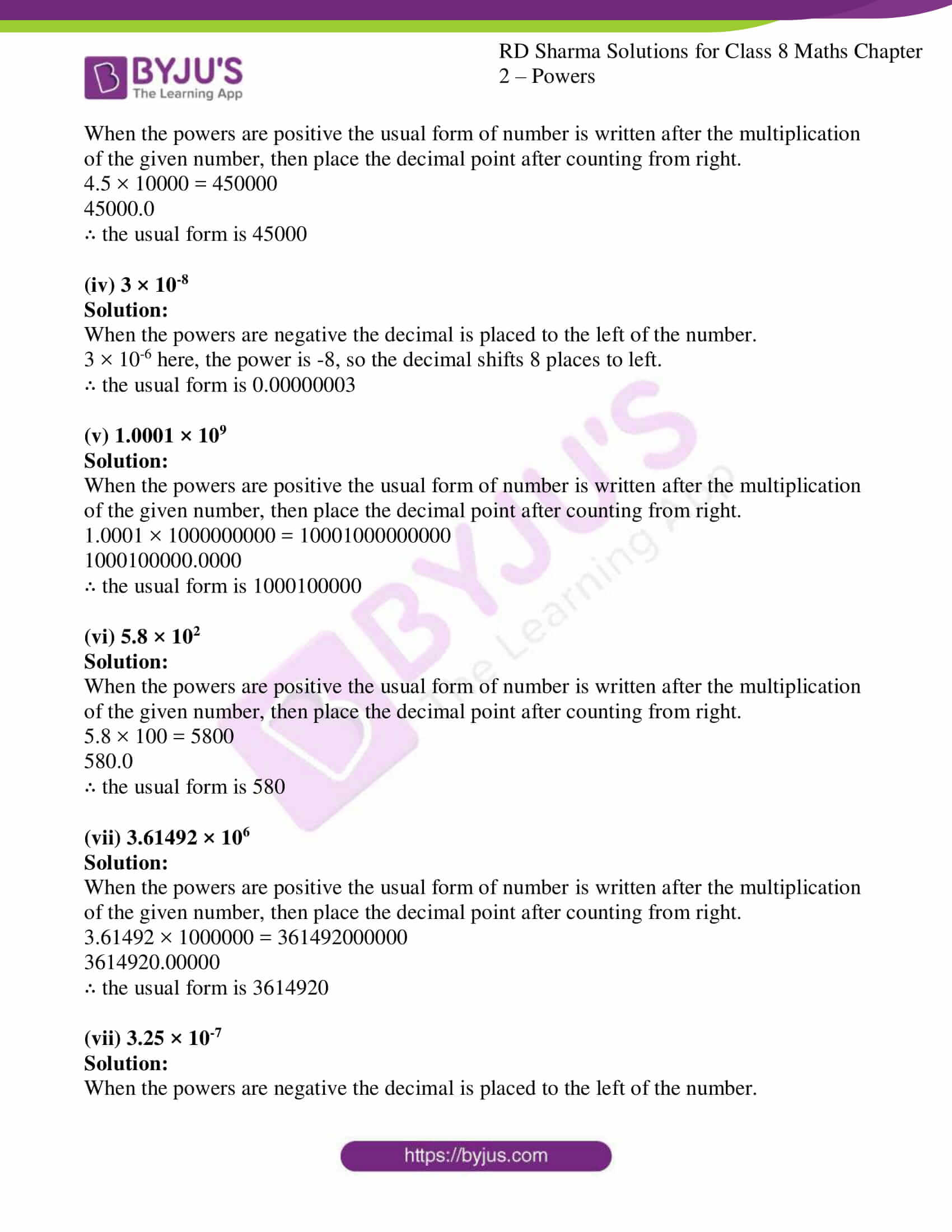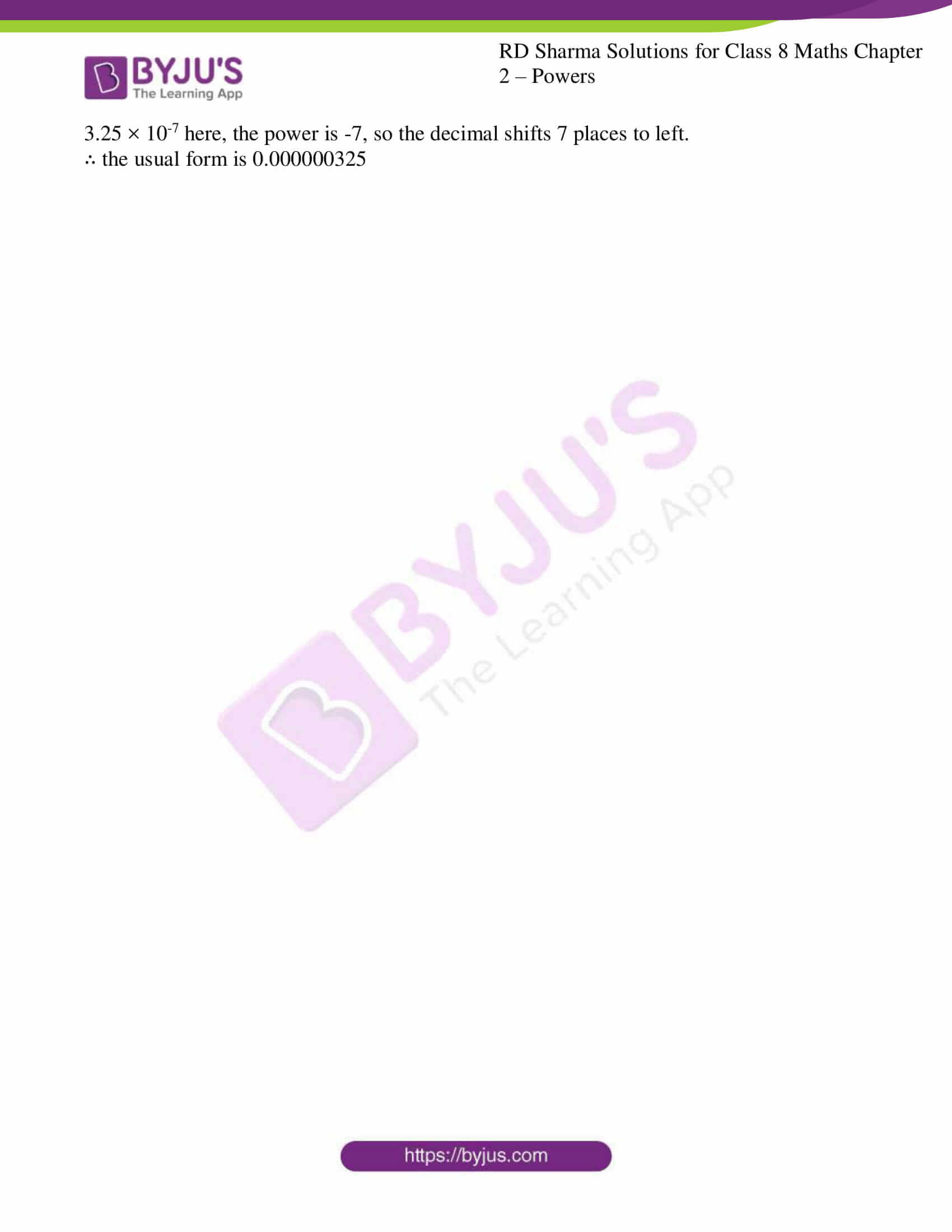## RD Sharma Solutions for Class 8 Maths Chapter 2 – Powers

Chapter 2- Powers contains 3 exercises and the RD Sharma Solutions present on this page provide the solutions for the questions present in each exercise.

## EXERCISE 2.1 PAGE NO: 2.8

1. Express each of the following as a rational number of the form p/q, where p and q are integers and q ≠ 0:

(i) 2-3

(ii) (-4)-2

(iii) 1/(3)-2

(iv) (1/2)-5

(v) (2/3)-2

Solution:

(i) 2-3 = 1/23 = 1/2×2×2 = 1/8 (we know that a-n = 1/an)

(ii) (-4)-2 = 1/-42 = 1/-4×-4 = 1/16 (we know that a-n = 1/an)

(iii) 1/(3)-2 = 32 = 3×3 = 9 (we know that 1/a-n = an)

(iv) (1/2)-5 = 25 / 15 = 2×2×2×2×2 = 32 (we know that a-n = 1/an)

(v) (2/3)-2 = 32 / 22 = 3×3 / 2×2 = 9/4 (we know that a-n = 1/an)

2. Find the values of each of the following:

(i) 3-1 + 4-1

(ii) (30 + 4-1) × 22

(iii) (3-1 + 4-1 + 5-1)0

(iv) ((1/3)-1 – (1/4)-1)-1

Solution:

(i) 3-1 + 4-1

1/3 + 1/4 (we know that a-n = 1/an)

LCM of 3 and 4 is 12

(1×4 + 1×3)/12

(4+3)/12

7/12

(ii) (30 + 4-1) × 22

(1 + 1/4) × 4 (we know that a-n = 1/an, a0 = 1)

LCM of 1 and 4 is 4

(1×4 + 1×1)/4 × 4

(4+1)/4 × 4

5/4 × 4

5

(iii) (3-1 + 4-1 + 5-1)0

(We know that a0 = 1)

(3-1 + 4-1 + 5-1)0 = 1

(iv) ((1/3)-1 – (1/4)-1)-1

(31 – 41)-1 (we know that 1/a-n = an, a-n = 1/an)

(3-4)-1

(-1)-1

1/-1 = -1

3. Find the values of each of the following:

(i) (1/2)-1 + (1/3)-1 + (1/4)-1

(ii) (1/2)-2 + (1/3)-2 + (1/4)-2

(iii) (2-1 × 4-1) ÷ 2-2

(iv) (5-1 × 2-1) ÷ 6-1

Solution:

(i) (1/2)-1 + (1/3)-1 + (1/4)-1

21 + 31 + 41 (we know that 1/a-n = an)

2+3+4 = 9

(ii) (1/2)-2 + (1/3)-2 + (1/4)-2

22 + 32 + 42 (we know that 1/a-n = an)

2×2 + 3×3 + 4×4

4+9+16 = 29

(iii) (2-1 × 4-1) ÷ 2-2

(1/21 × 1/41) / (1/22) (we know that a-n = 1/an)

(1/2 × 1/4) × 4/1 (we know that 1/a ÷ 1/b = 1/a × b/1)

1/2

(iv) (5-1 × 2-1) ÷ 6-1

(1/51 × 1/21) / (1/61) (we know that a-n = 1/an)

(1/5 × 1/2) × 6/1 (we know that 1/a ÷ 1/b = 1/a × b/1)

3/5

4. Simplify:

(i) (4-1 × 3-1)2

(ii) (5-1 ÷ 6-1)3

(iii) (2-1 + 3-1)-1

(iv) (3-1 × 4-1)-1 × 5-1

Solution:

(i) (4-1 × 3-1)2 (we know that a-n = 1/an)

(1/4 × 1/3)2

(1/12)2

(1×1 / 12×12)

1/144

(ii) (5-1 ÷ 6-1)3

((1/5) / (1/6))3 (we know that a-n = 1/an)

((1/5) × 6)3 (we know that 1/a ÷ 1/b = 1/a × b/1)

(6/5)3

6×6×6 / 5×5×5

216/125

(iii) (2-1 + 3-1)-1

(1/2 + 1/3)-1 (we know that a-n = 1/an)

LCM of 2 and 3 is 6

((1×3 + 1×2)/6)-1

(5/6)-1

6/5

(iv) (3-1 × 4-1)-1 × 5-1

(1/3 × 1/4)-1 × 1/5 (we know that a-n = 1/an)

(1/12)-1 × 1/5

12/5

5. Simplify:

(i) (32 + 22) × (1/2)3

(ii) (32 – 22) × (2/3)-3

(iii) ((1/3)-3 – (1/2)-3) ÷ (1/4)-3

(iv) (22 + 32 – 42) ÷ (3/2)2

Solution:

(i) (32 + 22) × (1/2)3

(9 + 4) × 1/8 = 13/8

(ii) (32 – 22) × (2/3)-3

(9-4) × (3/2)3

5 × (27/8)

135/8

(iii) ((1/3)-3 – (1/2)-3) ÷ (1/4)-3

(33 – 23) ÷ 43 (we know that 1/a-n = an)

(27-8) ÷ 64

19 × 1/64 (we know that 1/a ÷ 1/b = 1/a × b/1)

19/64

(iv) (22 + 32 – 42) ÷ (3/2)2

(4 + 9 – 16) ÷ (9/4)

(-3) × 4/9 (we know that 1/a ÷ 1/b = 1/a × b/1)

-4/3

6. By what number should 5-1 be multiplied so that the product may be equal to (-7)-1?

Solution:

Let us consider a number x

So, 5-1 × x = (-7)-1

1/5 × x = 1/-7

x = (-1/7) / (1/5)

= (-1/7) × (5/1)

= -5/7

7. By what number should (1/2)-1 be multiplied so that the product may be equal to (-4/7)-1?

Solution:

Let us consider a number x

So, (1/2)-1 × x = (-4/7)-1

1/(1/2) × x = 1/(-4/7)

x = (-7/4) / (2/1)

= (-7/4) × (1/2)

= -7/8

8. By what number should (-15)-1 be divided so that the quotient may be equal to (-5)-1?

Solution:

Let us consider a number x

So, (-15)-1 ÷ x = (-5)-1

1/-15 × 1/x = 1/-5

1/x = (1×-15)/-5

1/x = 3

x = 1/3

## EXERCISE 2.2 PAGE NO: 2.18

1. Write each of the following in exponential form:

(i) (3/2)-1 × (3/2)-1 × (3/2)-1 × (3/2)-1

(ii) (2/5)-2 × (2/5)-2 × (2/5)-2

Solution:

(i) (3/2)-1 × (3/2)-1 × (3/2)-1 × (3/2)-1

(3/2)-4 (we know that a-n = 1/an, an = a×a…n times)

(ii) (2/5)-2 × (2/5)-2 × (2/5)-2

(2/5)-6 (we know that a-n = 1/an, an = a×a…n times)

2. Evaluate:

(i) 5-2

(ii) (-3)-2

(iii) (1/3)-4

(iv) (-1/2)-1

Solution:

(i) 5-2

1/52 = 1/25 (we know that a-n = 1/an)

(ii) (-3)-2

(1/-3)2 = 1/9 (we know that a-n = 1/an)

(iii) (1/3)-4

34 = 81 (we know that 1/a-n = an)

(iv) (-1/2)-1

-21 = -2 (we know that 1/a-n = an)

3. Express each of the following as a rational number in the form p/q:

(i) 6-1

(ii) (-7)-1

(iii) (1/4)-1

(iv) (-4)-1 × (-3/2)-1

(v) (3/5)-1 × (5/2)-1

Solution:

(i) 6-1

1/61 = 1/6 (we know that a-n = 1/an)

(ii) (-7)-1

1/-71 = -1/7 (we know that a-n = 1/an)

(iii) (1/4)-1

41 = 4 (we know that 1/a-n = an)

(iv) (-4)-1 × (-3/2)-1

1/-41 × (2/-3)1 (we know that a-n = 1/an, 1/a-n = an)

1/-2 × -1/3

1/6

(v) (3/5)-1 × (5/2)-1

(5/3)1 × (2/5)1

5/3 × 2/5

2/3

4. Simplify:

(i) (4-1 × 3-1)2

(ii) (5-1 ÷ 6-1)3

(iii) (2-1 + 3-1)-1

(iv) (3-1 × 4-1)-1 × 5-1

(v) (4-1 – 5-1) ÷ 3-1

Solution:

(i) (4-1 × 3-1)2

(1/4 × 1/3)2 (we know that a-n = 1/an)

(1/12)2

1/144

(ii) (5-1 ÷ 6-1)3

(1/5 ÷ 1/6)3 (we know that a-n = 1/an)

(1/5 × 6)3 (we know that 1/a ÷ 1/b = 1/a × b/1)

(6/5)3

216/125

(iii) (2-1 + 3-1)-1

(1/2 + 1/3)-1 (we know that a-n = 1/an)

LCM of 2 and 3 is 6

((3+2)/6)-1

(5/6)-1 (we know that 1/a-n = an)

6/5

(iv) (3-1 × 4-1)-1 × 5-1

(1/3 × 1/4)-1 × 1/5 (we know that a-n = 1/an)

(1/12)-1 × 1/5 (we know that 1/a-n = an)

12 × 1/5

12/5

(v) (4-1 – 5-1) ÷ 3-1

(1/4 – 1/5) ÷ 1/3 (we know that a-n = 1/an)

LCM of 4 and 5 is 20

(5-4)/20 × 3/1 (we know that 1/a ÷ 1/b = 1/a × b/1)

1/20 × 3

3/20

5. Express each of the following rational numbers with a negative exponent:

(i) (1/4)3

(ii) 35

(iii) (3/5)4

(iv) ((3/2)4)-3

(v) ((7/3)4)-3

Solution:

(i) (1/4)3

(4)-3 (we know that 1/an = a-n)

(ii) 35

(1/3)-5 (we know that 1/an = a-n)

(iii) (3/5)4

(5/3)-4 (we know that (a/b)-n = (b/a)n)

(iv) ((3/2)4)-3

(3/2)-12 (we know that (an)m = anm)

(v) ((7/3)4)-3

(7/3)-12 (we know that (an)m = anm)

6. Express each of the following rational numbers with a positive exponent:

(i) (3/4)-2

(ii) (5/4)-3

(iii) 43 × 4-9

(iv) ((4/3)-3)-4

(v) ((3/2)4)-2

Solution:

(i) (3/4)-2

(4/3)2 (we know that (a/b)-n = (b/a)n)

(ii) (5/4)-3

(4/5)3 (we know that (a/b)-n = (b/a)n)

(iii) 43 × 4-9

(4)3-9 (we know that an × am = an+m)

4-6

(1/4)6 (we know that 1/an = a-n)

(iv) ((4/3)-3)-4

(4/3)12 (we know that (an)m = anm)

(v) ((3/2)4)-2

(3/2)-8 (we know that (an)m = anm)

(2/3)8 (we know that 1/an = a-n)

7. Simplify:

(i) ((1/3)-3 – (1/2)-3) ÷ (1/4)-3

(ii) (32 – 22) × (2/3)-3

(iii) ((1/2)-1 × (-4)-1)-1

(iv) (((-1/4)2)-2)-1

(v) ((2/3)2)3 × (1/3)-4 × 3-1 × 6-1

Solution:

(i) ((1/3)-3 – (1/2)-3) ÷ (1/4)-3

(33 – 23) ÷ 43 (we know that 1/an = a-n)

(27-8) ÷ 64

19 ÷ 64

19 × 1/64 (we know that 1/a ÷ 1/b = 1/a × b/1)

19/64

(ii) (32 – 22) × (2/3)-3

(9 – 4) × (3/2)3 (we know that 1/an = a-n)

5 × (27/8)

135/8

(iii) ((1/2)-1 × (-4)-1)-1

(21 × (1/-4))-1 (we know that 1/an = a-n)

(1/-2)-1 (we know that 1/an = a-n)

-21

-2

(iv) (((-1/4)2)-2)-1

((-1/16)-2)-1 (we know that 1/an = a-n)

((-16)2)-1 (we know that 1/an = a-n)

(256)-1 (we know that 1/an = a-n)

1/256

(v) ((2/3)2)3 × (1/3)-4 × 3-1 × 6-1

(4/9)3 × 34 × 1/3 × 1/6 (we know that 1/an = a-n)

(64/729) × 81 × 1/3 × 1/6

(64/729) × 27 × 1/6

32/729 × 27 × 1/3

32/729 × 9

32/81

8. By what number should 5-1 be multiplied so that the product may be equal to (-7)-1?

Solution:

Let us consider a number x

So, 5-1 × x = (-7)-1

1/5 × x = 1/-7 (we know that 1/an = a-n)

x = (-1/7) / (1/5)

= (-1/7) × (5/1) (we know that 1/a ÷ 1/b = 1/a × b/1)

= -5/7

9. By what number should (1/2)-1 be multiplied so that the product may be equal to (-4/7)-1?

Solution:

Let us consider a number x

So, (1/2)-1 × x = (-4/7)-1

1/(1/2) × x = 1/(-4/7) (we know that 1/an = a-n)

x = (-7/4) / (2/1)

= (-7/4) × (1/2) (we know that 1/a ÷ 1/b = 1/a × b/1)

= -7/8

10. By what number should (-15)-1 be divided so that the quotient may be equal to (-5)-1?

Solution:

Let us consider a number x

So, (-15)-1 ÷ x = (-5)-1 (we know that 1/a ÷ 1/b = 1/a × b/1)

1/-15 × 1/x = 1/-5 (we know that 1/an = a-n)

1/x = (1×-15)/-5

1/x = 3

x = 1/3

11. By what number should (5/3)-2 be multiplied so that the product may be (7/3)-1?

Solution:

Let us consider a number x

So, (5/3)-2 × x = (7/3)-1

1/(5/3)2 × x = 1/(7/3) (we know that 1/an = a-n)

x = (3/7) / (3/5)2

= (3/7) / (9/25)

= (3/7) × (25/9) (we know that 1/a ÷ 1/b = 1/a × b/1)

= (1/7) × (25/3)

= 25/21

12. Find x, if

(i) (1/4)-4 × (1/4)-8 = (1/4)-4x

Solution:

(1/4)-4 × (1/4)-8 = (1/4)-4x

(1/4)-4-8 = (1/4)-4x (we know that an × am = an+m)

(1/4)-12 = (1/4)-4x

When the bases are same we can directly equate the coefficients

-12 = -4x

x = -12/-4

= 3

(ii) (-1/2)-19 ÷ (-1/2)8 = (-1/2)-2x+1

Solution:

(-1/2)-19 ÷ (-1/2)8 = (-1/2)-2x+1

(1/2)-19-8 = (1/2)-2x+1 (we know that an ÷ am = an-m)

(1/2)-27 = (1/2)-2x+1

When the bases are same we can directly equate the coefficients

-27 = -2x+1

-2x = -27-1

x = -28/-2

= 14

(iii) (3/2)-3 × (3/2)5 = (3/2)2x+1

Solution:

(3/2)-3 × (3/2)5 = (3/2)2x+1

(3/2)-3+5 = (3/2)2x+1 (we know that an × am = an+m)

(3/2)2 = (3/2)2x+1

When the bases are same we can directly equate the coefficients

2 = 2x+1

2x = 2-1

x = 1/2

(iv) (2/5)-3 × (2/5)15 = (2/5)2+3x

Solution:

(2/5)-3 × (2/5)15 = (2/5)2+3x

(2/5)-3+15 = (2/5)2+3x (we know that an × am = an+m)

(2/5)12 = (2/5)2+3x

When the bases are same we can directly equate the coefficients

12 = 2+3x

3x = 12-2

x = 10/3

(v) (5/4)-x ÷ (5/4)-4 = (5/4)5

Solution:

(5/4)-x ÷ (5/4)-4 = (5/4)5

(5/4)-x+4 = (5/4)5 (we know that an ÷ am = an-m)

When the bases are same we can directly equate the coefficients

-x+4 = 5

-x = 5-4

-x = 1

x = -1

(vi) (8/3)2x+1 × (8/3)5 = (8/3) x+2

Solution:

(8/3)2x+1 × (8/3)5 = (8/3)x+2

(8/3)2x+1+5 = (8/3) x+2 (we know that an × am = an+m)

(8/3)2x+6 = (8/3) x+2

When the bases are same we can directly equate the coefficients

2x+6 = x+2

2x-x = -6+2

x = -4

13. (i) If x= (3/2)2 × (2/3)-4, find the value of x-2.

Solution:

x= (3/2)2 × (2/3)-4

= (3/2)2 × (3/2)4 (we know that 1/an = a-n)

= (3/2)2+4 (we know that an × am = an+m)

= (3/2)6

x-2 = ((3/2)6)-2

= (3/2)-12

= (2/3)12

(ii) If x = (4/5)-2 ÷ (1/4)2, find the value of x-1.

Solution:

x = (4/5)-2 ÷ (1/4)2

= (5/4)2 ÷ (1/4)2 (we know that 1/an = a-n)

= (5/4)2 × (4/1)2 (we know that 1/a ÷ 1/b = 1/a × b/1)

= 25/16 × 16

= 25

x-1 = 1/25

14. Find the value of x for which 52x ÷ 5-3 = 55

Solution:

52x ÷ 5-3 = 55

52x+3 = 55 (we know that an ÷ am = an-m)

When the bases are same we can directly equate the coefficients

2x+3 = 5

2x = 5-3

2x = 2

x = 1

## EXERCISE 2.3 PAGE NO: 2.22

1. Express the following numbers in standard form:

(i) 6020000000000000

Solution:

To express 6020000000000000 in standard form, count the total digits leaving 1st digit from the left. So the total number of digits becomes the power of 10. Therefore the decimal comes after the 1st digit.

the total digits leaving 1st digit from the left is 15

∴ the standard form is 6.02 × 1015

(ii) 0.00000000000942

Solution:

To express 0.00000000000942 in standard form,

Any number after the decimal point the powers become negative. Total digits after decimal is 12

∴ the standard form is 9.42 × 10-12

(iii) 0.00000000085

Solution:

To express 0.00000000085 in standard form,

Any number after the decimal point the powers become negative. Total digits after decimal is 10

∴ the standard form is 8.5 × 10-10

(iv) 846 × 107

Solution:

To express 846 × 107 in standard form, count the total digits leaving 1st digit from the left. So the total number of digits becomes the power of 10. Therefore the decimal comes after the 1st digit.

the total digits leaving 1st digit from the left is 2

846 × 107 = 8.46 × 102 × 107 = 8.46 × 102+7 = 8.46 × 109

(v) 3759 × 10-4

Solution:

To express 3759 × 10-4 in standard form, count the total digits leaving 1st digit from the left. So the total number of digits becomes the power of 10. Therefore the decimal comes after the 1st digit.

the total digits leaving 1st digit from the left is 3

3759 × 10-4 = 3.759 × 103 × 10-4 = 3.759 × 103+(-4) = 3.759 × 10-1

(vi) 0.00072984

Solution:

To express 0.00072984 in standard form,

Any number after the decimal point the powers become negative. Total digits after decimal is 4

∴ the standard form is 7.2984 × 10-4

(vii) 0.000437 × 104

Solution:

To express 0.000437 × 104 in standard form,

Any number after the decimal point the powers become negative. Total digits after decimal is 4

∴ the standard form is 4.37 × 10-4 × 104 = 4.37

(viii) 4 ÷ 100000

Solution:

To express in standard form count the number of zeros of the divisor. This count becomes the negative power of 10.

∴ the standard form is 4 × 10-5

2. Write the following numbers in the usual form:

(i) 4.83 × 107

Solution:

When the powers are positive the usual form of number is written after the multiplication of the given number, then place the decimal point after counting from right.

4.83 × 10000000 = 4830000000

48300000.00

∴ the usual form is 48300000

(ii) 3.02 × 10-6

Solution:

When the powers are negative the decimal is placed to the left of the number.

3.02 × 10-6 here, the power is -6, so the decimal shifts 6 places to left.

∴ the usual form is 0.00000302

(iii) 4.5 × 104

Solution:

When the powers are positive the usual form of number is written after the multiplication of the given number, then place the decimal point after counting from right.

4.5 × 10000 = 450000

45000.0

∴ the usual form is 45000

(iv) 3 × 10-8

Solution:

When the powers are negative the decimal is placed to the left of the number.

3 × 10-6 here, the power is -8, so the decimal shifts 8 places to left.

∴ the usual form is 0.00000003

(v) 1.0001 × 109

Solution:

When the powers are positive the usual form of number is written after the multiplication of the given number, then place the decimal point after counting from right.

1.0001 × 1000000000 = 10001000000000

1000100000.0000

∴ the usual form is 1000100000

(vi) 5.8 × 102

Solution:

When the powers are positive the usual form of number is written after the multiplication of the given number, then place the decimal point after counting from right.

5.8 × 100 = 5800

580.0

∴ the usual form is 580

(vii) 3.61492 × 106

Solution:

When the powers are positive the usual form of number is written after the multiplication of the given number, then place the decimal point after counting from right.

3.61492 × 1000000 = 361492000000

3614920.00000

∴ the usual form is 3614920

(vii) 3.25 × 10-7

Solution:

When the powers are negative the decimal is placed to the left of the number.

3.25 × 10-7 here, the power is -7, so the decimal shifts 7 places to left.

∴ the usual form is 0.000000325

### RD Sharma Solutions for Class 8 Maths Chapter 2 – Powers

Here students will be acquainted with detailed concepts discussed in this Chapter as listed below.

• Integral exponents – Here we will learn about different types of exponents (Zero exponents, Positive Exponents, Negative Exponents, etc) right from the fundamental way of adding and subtracting the numbers with the same power or the same numbers having different powers.
• Decimal number system.
• Laws of integral exponents.
• Use of exponents to express small numbers in standard form.